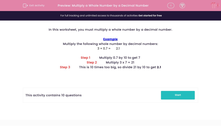# Multiply a Whole Number by a Decimal Number

In this worksheet, students multiply a whole number by a simple decimal number.Key stage:  KS 2

Curriculum topic:   Maths and Numerical Reasoning

Curriculum subtopic:   Decimals

Difficulty level:#### Worksheet Overview

In this worksheet, you must multiply a whole number by a decimal number.

Example

Multiply the following whole number by decimal numbers:

3 × 0.7 =
 2.1

Step 1          Multiply 0.7 by 10 to get 7

Step 2          Multiply 3 x 7 = 21

Step 3          This is 10 times too big, so divide 21 by 10 to get 2.1

### What is EdPlace?

We're your National Curriculum aligned online education content provider helping each child succeed in English, maths and science from year 1 to GCSE. With an EdPlace account you’ll be able to track and measure progress, helping each child achieve their best. We build confidence and attainment by personalising each child’s learning at a level that suits them.

Get started